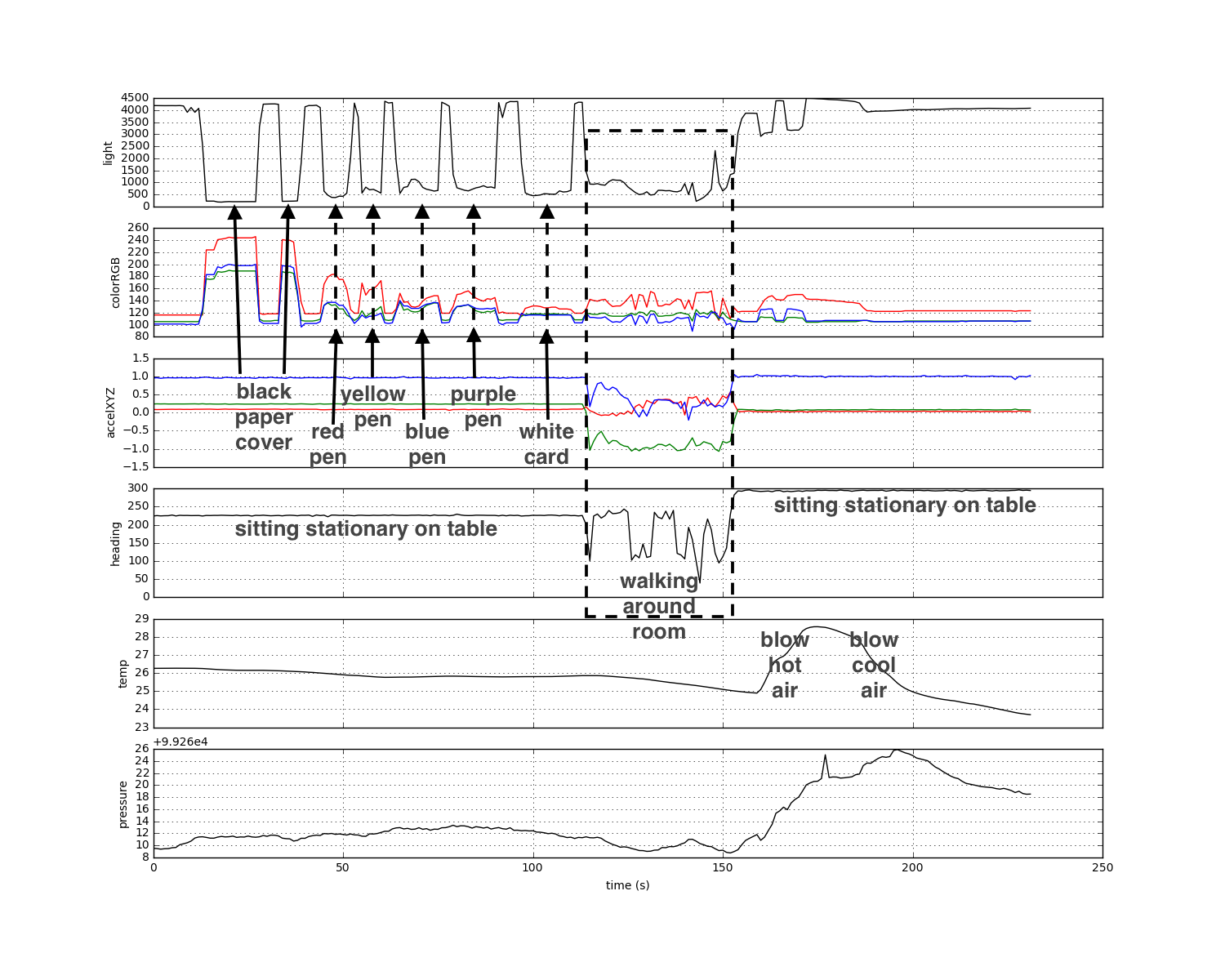## Explorations of Pimoroni's Enviro pHAT sensor HAT on a Raspberry Pi Zero

### RPi on battery running a Jupyter notebook server, accessed remotely via wifi

Andrew Ganse, http://research.ganse.org

import time
from envirophat import light, motion, weather, leds

# device laying on table
print light.light()
print light.rgb()
print weather.temperature()
print weather.pressure()
print motion.accelerometer()

4207
(120, 108, 103)
26.0951736262
99278.0698014
(0.08953857421875, 0.24188232421875, 0.957275390625)
223.83

# device laying on table with heavy sheet paper over it
print light.light()
print light.rgb()
print weather.temperature()
print weather.pressure()
print motion.accelerometer()

307
(225, 179, 186)
26.0460905325
99277.2708534
(0.09661865234375, 0.241455078125, 0.96038818359375)
224.47

out = open('enviro.log', 'w')

try:
while True:
lux = light.light()
leds.on()
rgb = light.rgb()
leds.off()
acc = motion.accelerometer()
temp = weather.temperature()
press = weather.pressure()
print('%d %d %d %d %f %f %f %f %f %f' % \
(lux, rgb, rgb, rgb, acc, acc, acc, heading, temp, press))
out.write('%d %d %d %d %f %f %f %f %f %f\n' % \
(lux, rgb, rgb, rgb, acc, acc, acc, heading, temp, press))
time.sleep(1)  # (set to "time.sleep(0.1)" for second run)

except KeyboardInterrupt:
leds.off()
out.close()

3744 121 103 103 -0.019531 0.085449 1.002563 305.720000 24.042208 99279.639506
3744 121 103 103 -0.022339 0.086243 1.003113 306.690000 24.040343 99279.521707
3744 121 103 103 -0.023682 0.083069 1.003784 304.800000 24.040343 99279.521707
3744 121 103 103 -0.021362 0.086121 0.998962 305.500000 24.040343 99279.521707
...

import csv
data_file = open('enviro.log')

lux=[]; colorR=[]; colorG=[]; colorB=[]
accX=[]; accY=[]; accZ=[]; heading=[]; temp=[]; press=[];
for row in file_reader:
lux.append(row)
colorR.append(row)
colorG.append(row)
colorB.append(row)
accX.append(row)
accY.append(row)
accZ.append(row)
temp.append(row)
press.append(row)

import matplotlib.pyplot as plt
%matplotlib inline
f, ax = plt.subplots(6, sharex=True, figsize=(15, 12))

ax.plot(lux,'k')
ax.set_ylabel('light')
ax.grid(True)
ax.plot(colorR,'r')
ax.plot(colorG,'g')
ax.plot(colorB,'b')
ax.set_ylabel('colorRGB')
ax.grid(True)
ax.plot(accX,'r')
ax.plot(accY,'g')
ax.plot(accZ,'b')
ax.set_ylabel('accelXYZ')
ax.grid(True)
ax.grid(True)
ax.plot(temp,'k')
ax.set_ylabel('temp')
ax.grid(True)
ax.plot(press,'k')
ax.set_ylabel('pressure')
ax.grid(True)
ax.set_xlabel('time (s)')  # sample rate was 1sec
f.savefig("data.png")# second dataset focusing on acccelerations in 3 axis directions...
# (note this run was done with sample rate 10 Hz)
import numpy as np
f, ax = plt.subplots(1, figsize=(15, 3))
t=np.arange(0,float(len(accX))/10.0,0.1)
ax.plot(t,accX,'r.-')
ax.plot(t,accY,'g.-')
ax.plot(t,accZ,'b.-')
ax.set_ylabel('accelXYZ')
ax.grid(True)
ax.set_xlabel('time (s)')  # sample rate was 1sec
f.savefig("data2.png")With roughly 1Hz period, I first shook the device vertically up and down about 5-6 times, then left/right about 5-6 times, then fwd/back 5-6 times. We can see the three directions reasonably well in the data, given the caveat that I had no idea which directions were the device's unit directions and wasn't be very careful anyway. Note for this run I increased the sampling rate to 10Hz to catch my roughly 1Hz shaking period.